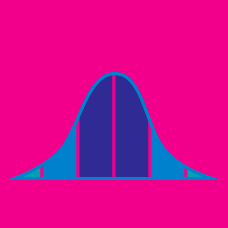Probability

# Covariance

If the joint probability distribution function of $X$ and $Y$ is given as follows, what is $20 \text{Cov}(X,Y) ?$

$\begin{matrix} p(X,Y) & X=0 & X=1 & X=2 \\ Y=0 & 0.1 & 0.1 & 0.2 \\ Y=1 & 0.2 & 0.2 & 0 \\ Y=2 & 0 & 0.1 & 0.1 \end{matrix}$

For random variables $X$ and $Y,$ which of the following is the same as $\text{Cov}(X,Y)?$

If the joint probability density function of continuous random variables $X$ and $Y$ is $f(x,y) = \frac{1}{6} \left( 8 x + 4 y \right)$ with $0 \le x \le 1 \text{ and } 0 \le y \le 1,$ what is the covariance between $X$ and $Y?$

If $X$ and $Y$ are independent random variables where $E[X]=10$ and $E[Y]=8,$ what is $E[XY]?$

If the probability distributions of continuous random variables $X$ and $Y$ are independent of each other, which of the following is equal to $\text{Cov}(X,Y)?$

×

Problem Loading...

Note Loading...

Set Loading...